GET THE APP

Estimation of Some Thermal Diffusion Parameters by Coupling the Fractional Derivative Theory and the Genetic Algorithm

# Journal of Applied & Computational Mathematics

ISSN: 2168-9679

Open Access
Submit Manuscript arrow_forward arrow_forwardResearch Article - (2021) Volume 10, Issue 12

# Estimation of Some Thermal Diffusion Parameters by Coupling the Fractional Derivative Theory and the Genetic Algorithm

Rasolomampiandry G1*, Rakotoson R2 and Randimbindrainibe F3
*Correspondence: Rasolomampiandry G, Cognitive Sciences and Applications Research Laboratory, BP 1500, Ankatso - Antananarivo 101, Madagascar, Email:
1Cognitive Sciences and Applications Research Laboratory (LR - SCA), Madagascar
2Doctoral School in Engineering and Innovation Sciences and Techniques (ED - STII), Madagascar
3Ecole Supérieure Polytechnique Antananarivo (ESPA) - University of Antananarivo, BP 1500, Ankatso - Antananarivo 101, Madagascar

Citation: Rasolomampiandry G, Rakotoson R, Randimbindrainibe F. "Estimation of Some Thermal Diffusion Parameters by Coupling the Fractional Derivative Theory and the Genetic Algorithm." J Appl Computat Math 9 (2021): 498.
Copyright: © 2021 Rasolomampiandry G. This is an open-access article distributed under the terms of the Creative Commons Attribution License, which permits unrestricted use, distribution, and reproduction in any medium, provided the original author and source are credited.

## Abstract

This work consists in calculating values of thermal conductivity and the suitable thickness, of a thermal insulator called "thermisorel", by the coupling of the time fractional diffusion equation and the genetic algorithm, taking into account the data and the objectives of the experiment in the article .

#### Keywords

Genetic algorithm • Thermisorel

#### Introduction

In , a heat transfer study was performed on a sample of low density wood fiber board, called Thermisorel. This material is manufactured by STEICO Casteljaloux in France. Thermisorel is used in construction because it prevents heat loss in winter due to their low thermal conductivity, nearly 0.042 Wm-1 K-1. It also protects the building from heat in summer due to their capacity high thermal storage. In this study, a 22.2 mm thick thermisorel board was used. The details of this study are described . Only the data necessary for our problem mentioned above, which we will recall. This work will be divided into two parts:

In section 2, we will detail the position of our problem, as well as the hypotheses necessary for its resolution. In the third part, we will talk about the genetic algorithm, as well as the time fractional diffusion equation and its discretization in order to give the desired solution

Positioning of the problem

Our problem is to identify suitable optimal values of thermal conductivity and thickness of a thermal insulator, if we vary from 15°C to 24.6°C the temperature of one of the faces and the other will keep a constant temperature of 15°. To solve this problem, we will use as hypotheses, the data used from the experiment in :

- The density of the insulation: ρ =170kg.m−3

- Specific heat: Cp =1280 J kg K−1 (according to the measurement made by the CSBT)

- Temperature and heat flux variations as a function of time, on both sides of the thermal insulator plate (see tables below)

From the graphs in , we extracted the values of the temperature and those of the flux as a function of time, until the steady state was reached, for the hot side. We have the following tables (Tables 1-3):

- Since the temperature and the heat flux at any point of each face, at a given time, are identical, then we can restrict the study to a straight segment perpendicular to the two faces, one of the ends of which (noted O) on hot face and the other (noted X) on the face where the temperature is constant

- It is assumed that the variation of the flux, at a given moment, on this segment is linear

The problem thus turns to the following question:

What are the optimal values suitable for thermal conductivity and segment length [O, X]? If the temperature at point O varies according to Table 1,2&3 during the transient period. And that the temperature of the X end is 15°C during the two regimes, permanent and stationary.

Table 1: Table representation of the temperature variation of the hot face during the transient regime.

Time in seconds Temperature in °C
0 15
10 15
20 15.67
30 16.34
40 17.34
50 17.5
60 18
70 19
80 20
90 21
100 22.34
110 23.67
120 24.33
130 24 .66

Table 2: Table representation of the variation of the flux of the hot face during the transient regime.

Time in seconds Flux in W .m-2
0 0
10 0
20 18.34
30 20
40 30
50 33.33
60 33.34
70 38.34
80 41 .67
90 46 .67
100 43.34
110 38.34
120 31.67
130 18 .37

Table 3: Representation in table form of the variation of the flow of the face where the temperature remains constant during the transient regime.

Time in seconds Flux in W .m-2
0 0
10 0
20 0
30 0
40 0
50 0
60 0.77
70 1.54
80 2.31
90 4.62
100 4.62
110 7.69
120 9.23
130 10.77

The solution is therefore the values of these two parameters which can give at point X the temperature value closest to 15°C. (If we use the means mentioned at the beginning)

Solution of the Problem by Coupling the Genetic Algorithm and the Fractional Diffusion Equation

The time fractional diffusion equation

In the case of this problem the time fractional diffusion equation is written: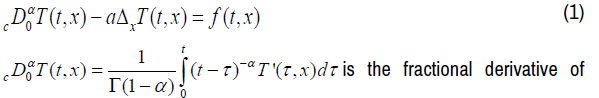Caputo of order α, 0 <α <1 of the variable t, t> 0 [2,3]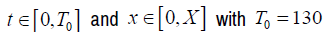be determined and which corresponds to the thickness of the thermal insulator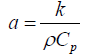is the diffusivity coefficient. kis the other parameter to be determined

T(t, x) is the temperature corresponding to the variables t and x

f (t, x) is the flow corresponding to the variables t and x

For our discretization:

Note that the transient regime stops at the 130th second. Then on the segment [0,T0 ]

And [0, X ] , we respectively construct a finite sequence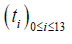such thatand ti = il , a finite sequence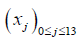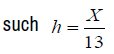that and xj = jh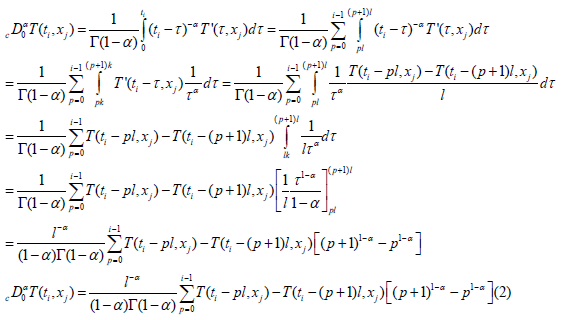This relation is valid for i ≠ 0

For the Laplacian discretization we will use the advanced decentered differentiation  and we have: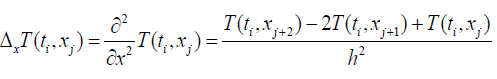(3)

The linearity assumption on f (t, x) allows us to write: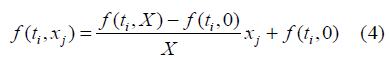Equation (1) has become:

To simplify the writing, note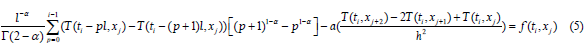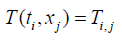hence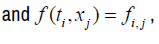and the équation (5) has become: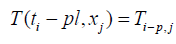We further assume that for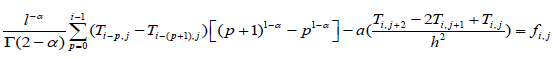For i =1 et 1≤ j ≤13 , (5) is written: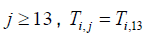Specifically

For i = 1 and j = 12 we have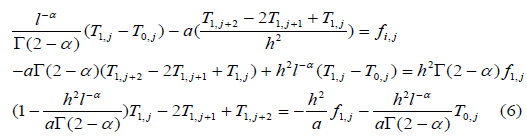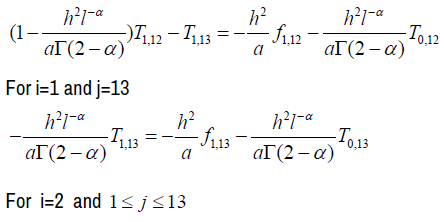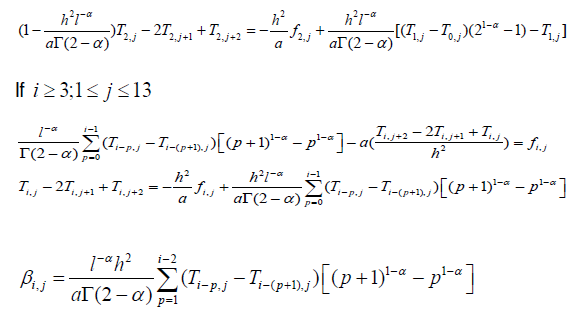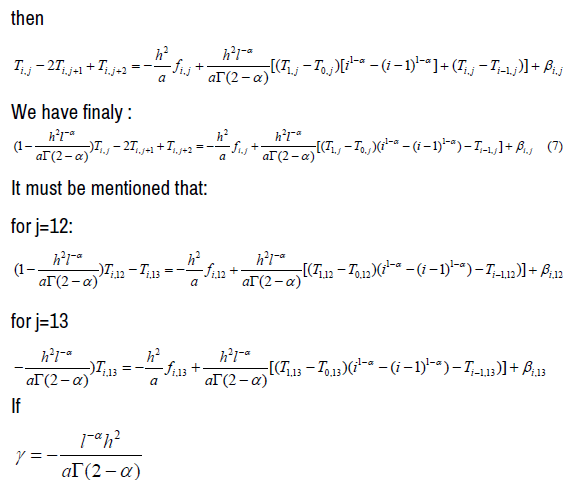We deduce the following matrix forms:

For i =1;1≤ j ≤13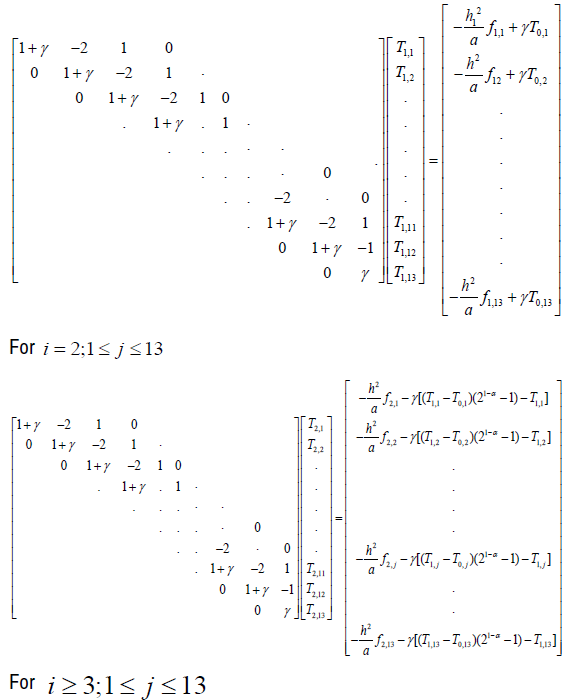We then have 13 matrix equations of the form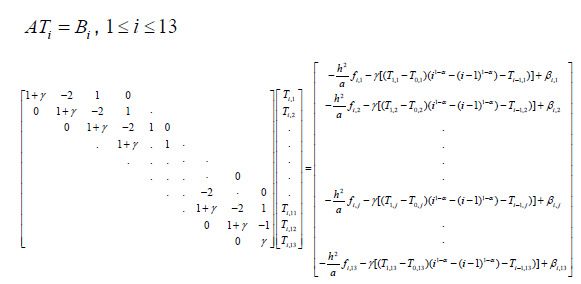If we fix kc and X, the solution of these equations following the values of i , gives

us a value of T(13,13) , when i=13 and which the value of the temperature

at the point X . If T(13,13) =15°C then kc and X are the solutions of the problem posed. Otherwise we change the value of kc and X. We only stop if T(13,13) =15°C

To speed up the search of kc and X, we set the search intervals for the two parameters and by means of the genetic algorithm, we can give optimal values, which depends on the imposed acceptance

The genetic algorithm

For the classical method, to identify a parameter θ , suitable for a given problem, in a set A, we consider a finite sequence of elements of A and among these elements we look for what respects the imposed conditions. If none of these elements is valid, we change immediately until we have an optimal solution. This process is long and can lead to a lot of wasted time in some cases .

Regarding the genetic algorithm, the choice of θ is made directly in A, even if A is uncountable; everything is predefined to avoid wasting time

Optimal values of X and K C

If α = 0.9 is the order of the fractional derivative. If we choose [0.03;0.045] c k ∈ and X ∈[0.02;0.03] , the computation with MATLAB, by means of the genetic algorithm, gives us the optimal solution: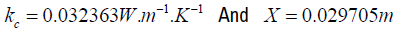. There is a difference between the values pronounced during the experiment and the values found by means of the genetic algorithm. But if we use as a hypothesis these theoretical values in  for example, we arrive at the same result.

#### Conclusion

In this article, we made an example of the coupling of a fractional differential equation and the genetic algorithm to optimize certain physical parameters. In future publications we will consider yet another fractional differential equation suitable for another physical system and which we will couple with an algorithm, such as what we used to optimize certain parameters.

#### Journal Highlights

###### Citations: 1282

Journal of Applied & Computational Mathematics received 1282 citations as per Google Scholar report